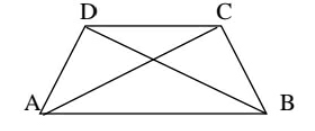"
">

# In the figure given below .Is \$ A B+B C+C D+D Aanswer."

Solution:

Let the diagonals AC and BD intersect in point O.

Since the sum of lengths of any two sides in a triangle should be greater than the length of the third side. Therefore,

In Δ AOB, AB < OA + OB ……….(i)

In Δ BOC, BC < OB + OC ……….(ii)

In Δ COD, CD < OC + OD ……….(iii)

In Δ AOD, DA < OD + OA ……….(iv)

⇒ AB + BC + CD + DA < 2OA + 2OB + 2OC +

2OD  ⇒ AB + BC + CD + DA < 2[(AO + OC) + (DO

+ OB)]  ⇒ AB + BC + CD + DA < 2(AC + BD)

Hence, it is proved.

Updated on: 10-Oct-2022

23 Views